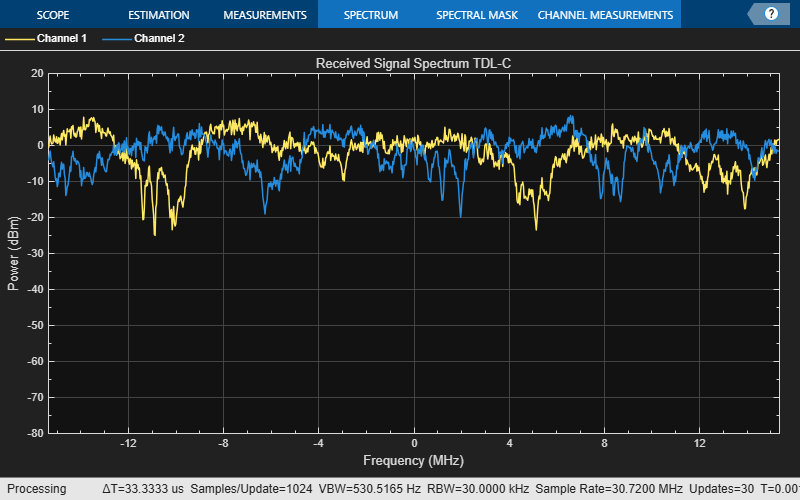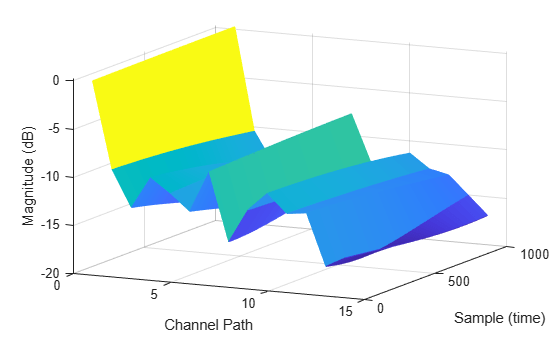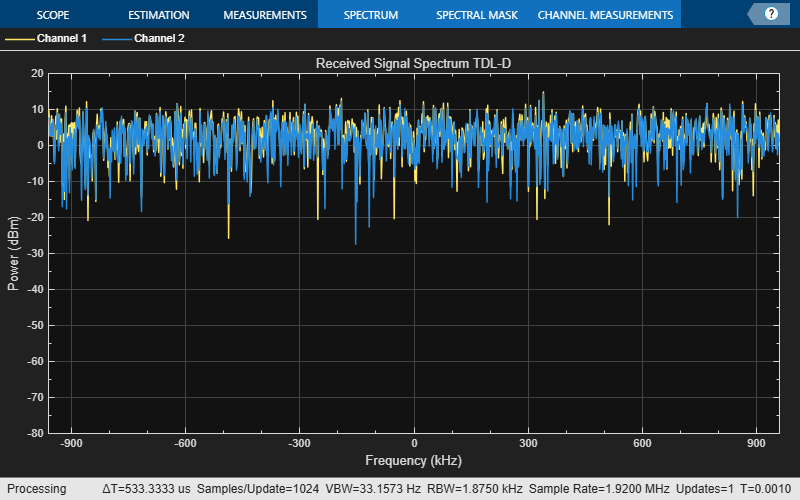# nrTDLChannel

Send signal through TDL channel model

## Description

The `nrTDLChannel` System object™ sends an input signal through a tapped delay line (TDL) multi-input multi-output (MIMO) link-level fading channel to obtain the channel-impaired signal. The object implements the following aspects of TR 38.901 :

• Section 7.7.2: TDL models

• Section 7.7.3: Scaling of delays

• Section 7.7.5.2 TDL extension: Applying a correlation matrix

• Section 7.7.6: K-factor for LOS channel models

To send a signal through the TDL MIMO channel model:

1. Create the `nrTDLChannel` object and set its properties.

2. Call the object with arguments, as if it were a function.

## Creation

### Syntax

``tdl = nrTDLChannel``
``tdl = nrTDLChannel(Name,Value)``

### Description

````tdl = nrTDLChannel` creates a TDL MIMO channel System object.```
````tdl = nrTDLChannel(Name,Value)` creates the object with properties set by using one or more name-value pairs. Enclose the property name inside quotes, followed by the specified value. Unspecified properties take default values.Example: ```tdl = nrTDLChannel('DelayProfile','TDL-D','DelaySpread',2e-6)``` creates a TDL channel model with TDL-D delay profile and a 2-microseconds delay spread.```

## Properties

expand all

Unless otherwise indicated, properties are nontunable, which means you cannot change their values after calling the object. Objects lock when you call them, and the `release` function unlocks them.

If a property is tunable, you can change its value at any time.

TDL delay profile, specified as one of `'TDL-A'`, `'TDL-B'`, `'TDL-C'`, `'TDL-D'`, `'TDL-E'`, or `'Custom'`. See TR 38.901 Section 7.7.2, Tables 7.7.2-1 to 7.7.2-5.

When you set this property to `'Custom'`, configure the delay profile using properties `PathDelays`, `AveragePathGains`, `FadingDistribution`, and `KFactorFirstTap`.

Data Types: `char` | `string`

Discrete path delays in seconds, specified as a numeric scalar or row vector. `AveragePathGains` and `PathDelays` must have the same size.

#### Dependencies

To enable this property, set `DelayProfile` to `'Custom'`.

Data Types: `double`

Average path gains in dB, specified as a numeric scalar or row vector. `AveragePathGains` and `PathDelays` must have the same size.

#### Dependencies

To enable this property, set `DelayProfile` to `'Custom'`.

Data Types: `double`

Fading process statistical distribution, specified as `'Rayleigh'` or `'Rician'`.

#### Dependencies

To enable this property, set `DelayProfile` to `'Custom'`.

Data Types: `char` | `string`

K-factor of first tap of delay profile in dB, specified as a numerical scalar. The default value corresponds to the K-factor of the first tap of TDL-D as defined in TR 38.901 Section 7.7.2, Table 7.7.2-4.

#### Dependencies

To enable this property, set `DelayProfile` to `'Custom'` and `FadingDistribution` to `'Rician'`.

Data Types: `double`

Desired root mean square (RMS) delay spread in seconds, specified as a numeric scalar. For examples of desired RMS delay spreads, `DSdesired`, see TR 38.901 Section 7.7.3 and Tables 7.7.3-1 and 7.7.3-2.

#### Dependencies

To enable this property, set `DelayProfile` to `'TDL-A'`, `'TDL-B'`, `'TDL-C'`, `'TDL-D'`, or `'TDL-E'`. This property does not apply for custom delay profile.

Data Types: `double`

Maximum Doppler shift in Hz, specified as a nonnegative numeric scalar. This property applies to all channel paths. When the maximum Doppler shift is set to 0, the channel remains static for the entire input. To generate a new channel realization, reset the object by calling the `reset` function.

Data Types: `double`

K-factor scaling, specified as `false` or `true`. When set to `true`, the `KFactor` property specifies the desired K-factor, and the object applies K-factor scaling as described in TR 38.901 Section 7.7.6.

### Note

K-factor scaling modifies both the path delays and path powers.

#### Dependencies

To enable this property, set `DelayProfile` to `'TDL-D'` or `'TDL-E'`.

Data Types: `double`

Desired K-factor for scaling in dB, specified as a numeric scalar. For typical K-factor values, see TR 38.901 Section 7.7.6 and Table 7.5-6.

### Note

• K-factor scaling modifies both the path delays and path powers.

• `K-factor` applies to the overall delay profile. Specifically, the K-factor after the scaling is `Kmodel` as described in TR 38.901 Section 7.7.6. `Kmodel` is the ratio of the power of the first path LOS to the total power of all the Rayleigh paths, including the Rayleigh part of the first path.

#### Dependencies

To enable this property, set `KFactorScaling` to `true`.

Data Types: `double`

Sample rate of input signal in Hz, specified as a positive numeric scalar.

Data Types: `double`

Correlation between user equipment (UE) and base station (BS) antennas, specified as one of these values:

• `'Low'` or `'High'` — Applies to both uplink and downlink. `'Low'` is equivalent to no correlation between antennas.

• `'Medium'` or `'Medium-A'` — For downlink, see TS 36.101 Annex B.2.3.2. For uplink, see TS 36.104 Annex B.5.2. The `TransmissionDirection` property controls the transmission direction.

• `'UplinkMedium'` — See TS 36.104, Annex B.5.2.

• `'Custom'` — The `ReceiveCorrelationMatrix` property specifies the correlation between UE antennas, and the `TransmitCorrelationMatrix` property specifies the correlation between BS antennas. See TR 38.901 Section 7.7.5.2.

For more details on correlation between UE and BS antennas, see TS 36.101  and TS 36.104 

Data Types: `char` | `string`

Antenna polarization arrangement, specified as `'Co-Polar'`, `'Cross-Polar'`, `'Custom'`.

Data Types: `char` | `string`

Transmission direction, specified as `'Downlink'` or `'Uplink'`.

#### Dependencies

To enable this property, set `MIMOCorrelation` to `'Low'`, `'Medium'`, `'Medium-A'`, `'UplinkMedium'`, or `'High'`.

Data Types: `char` | `string`

Number of transmit antennas, specified as a positive integer.

#### Dependencies

To enable this property, set `MIMOCorrelation` to `'Low'`, `'Medium'`, `'Medium-A'`, `'UplinkMedium'`, or `'High'`, or set both `MIMOCorrelation` and `Polarization` to `'Custom'`.

Data Types: `double`

Number of receive antennas, specified as a positive integer.

#### Dependencies

To enable this property, set `MIMOCorrelation` to `'Low'`, `'Medium'`, `'Medium-A'`, `'UplinkMedium'`, or `'High'`.

Data Types: `double`

Spatial correlation of transmitter, specified as a 2-D matrix or 3-D array.

• If the channel is frequency-flat (`PathDelays` is a scalar), specify `TransmitCorrelationMatrix` as a 2-D Hermitian matrix of size NT-by-NT. NT is the number of transmit antennas. The main diagonal elements must be all ones, and the off-diagonal elements must have a magnitude smaller than or equal to one.

• If the channel is frequency-selective (`PathDelays` is a row vector of length NP), specify `TransmitCorrelationMatrix` as one of these arrays:

• 2-D Hermitian matrix of size NT-by-NT with element properties as previously described. Each path has the same transmit correlation matrix.

• 3-D array of size NT-by-NT-by-NP, where each submatrix of size NT-by-NT is a Hermitian matrix with element properties as previously described. Each path has its own transmit correlation matrix.

#### Dependencies

To enable this property, set `MIMOCorrelation` to `'Custom'` and `Polarization` to either `'Co-Polar'` or `'Cross-Polar'`.

Data Types: `double`
Complex Number Support: Yes

Spatial correlation of receiver, specified as a 2-D matrix or 3-D array.

• If the channel is frequency-flat (`PathDelays` is a scalar), specify `ReceiveCorrelationMatrix` as a 2-D Hermitian matrix of size NR-by-NR. NR is the number of receive antennas. The main diagonal elements must be all ones, and the off-diagonal elements must have a magnitude smaller than or equal to one.

• If the channel is frequency-selective (`PathDelays` is a row vector of length NP), specify `ReceiveCorrelationMatrix` as one of these arrays:

• 2-D Hermitian matrix of size NR-by-NR with element properties as previously described. Each path has the same receive correlation matrix.

• 3-D array of size NR-by-NR-by-NP, where each submatrix of size NR-by-NR is a Hermitian matrix with element properties as previously described. Each path has its own receive correlation matrix.

#### Dependencies

To enable this property, set `MIMOCorrelation` to `'Custom'` and `Polarization` to either `'Co-Polar'` or `'Cross-Polar'`.

Data Types: `double`
Complex Number Support: Yes

Transmit polarization slant angles in degrees, specified as a row vector.

#### Dependencies

To enable this property, set `MIMOCorrelation` to `'Custom'` and `Polarization` to `'Cross-Polar'`.

Data Types: `double`

Receive polarization slant angles in degrees, specified as a row vector.

#### Dependencies

To enable this property, set `MIMOCorrelation` to `'Custom'` and `Polarization` to `'Cross-Polar'`.

Data Types: `double`

Cross-polarization power ratio in dB, specified as a numeric scalar or a row vector. This property corresponds to the ratio between the vertical-to-vertical (PVV) and vertical-to-horizontal (PVH) polarizations defined for the clustered delay line (CDL) models in TR 38.901 Section 7.7.1.

• If the channel is frequency-flat (`PathDelays` is a scalar), specify `XPR` as a scalar.

• If the channel is frequency-selective (`PathDelays` is a row vector of length NP), specify `XPR` as one of these values:

• Scalar — Each path has the same cross-polarization power ratio.

• Row vector of size 1-by-NP — Each path has its own cross-polarization power ratio.

The default value corresponds to the cluster-wise cross-polarization power ratio of CDL-A as defined in TR 38.901 Section 7.7.1, Table 7.7.1-1.

#### Dependencies

To enable this property, set `MIMOCorrelation` to `'Custom'` and `Polarization` to `'Cross-Polar'`.

Data Types: `double`

Combined correlation for the channel, specified as 2-D matrix or 3-D array. The matrix determines the product of the number of transmit antennas (NT) and the number of receive antennas (NR).

• If the channel is frequency-flat (`PathDelays` is a scalar), specify `SpatialCorrelationMatrix` as a 2-D Hermitian matrix of size (NTNR)-by-(NTNR).The magnitude of any off-diagonal element must be no larger than the geometric mean of the two corresponding diagonal elements.

• If the channel is frequency-selective (`PathDelays` is a row vector of length NP), specify `SpatialCorrelationMatrix` as one of these arrays:

• 2-D Hermitian matrix of size (NTNR)-by-(NTNR) with off-diagonal element properties as previously described. Each path has the same spatial correlation matrix.

• 3-D array of size (NTNR)-by-(NTNR)-by-NP array — where each matrix of size (NTNR)-by-(NTNR) is a Hermitian matrix with off-diagonal element properties as previously described. Each path has its own spatial correlation matrix.

#### Dependencies

To enable this property, set `MIMOCorrelation` to `'Custom'` and `Polarization` to `'Custom'`.

Data Types: `double`

Normalize path gains, specified as `true` or `false`. Use this property to normalize the fading processes. When this property is set to `true`, the total power of the path gains, averaged over time, is 0 dB. When this property is set to `false`, the path gains are not normalized. The average powers of the path gains are specified by the selected delay profile, or if `DelayProfile` is set to `'Custom'`, by the `AveragePathGains` property.

Data Types: `logical`

Time offset of fading process in seconds, specified as a numeric scalar.

Tunable: Yes

Data Types: `double`

Number of modeling sinusoids, specified as a positive integer. These sinusoids model the fading process.

Data Types: `double`

Source of random number stream, specified as one of the following:

• `'mt19937ar with seed'` — The object uses the mt19937ar algorithm for normally distributed random number generation. Calling the `reset` function resets the filters and reinitializes the random number stream to the value of the `Seed` property.

• `'Global stream'` — The object uses the current global random number stream for normally distributed random number generation. Calling the `reset` function resets only the filters.

Initial seed of mt19937ar random number stream, specified as a nonnegative numeric scalar.

#### Dependencies

To enable this property, set RandomStream to `'mt19937ar with seed'`. When calling the `reset` function, the seed reinitializes the mt19937ar random number stream.

Data Types: `double`

Normalize channel outputs by the number of receive antennas, specified as `true` or `false`.

Data Types: `logical`

## Usage

### Syntax

``signalOut = tdl(signalIn)``
``[signalOut,pathGains] = tdl(signalIn)``
``[signalOut,pathGains,sampleTimes] = tdl(signalIn)``

### Description

example

````signalOut = tdl(signalIn)` sends the input signal through a TDL MIMO fading channel and returns the channel-impaired signal.```

example

````[signalOut,pathGains] = tdl(signalIn)` also returns the MIMO channel path gains of the underlying fading process.```
````[signalOut,pathGains,sampleTimes] = tdl(signalIn)` also returns the sample times of the channel snapshots of the path gains.```

### Input Arguments

expand all

Input signal, specified as a complex scalar, vector, or NS-by-NT matrix, where:

• NS is the number of samples.

• NT is the number of transmit antennas.

Data Types: `single` | `double`
Complex Number Support: Yes

### Output Arguments

expand all

Output signal, returned as a complex scalar, vector, or NS-by-NR matrix, where:

• NS is the number of samples.

• NR is the number of receive antennas.

The output signal data type is of the same precision as the input signal data type.

Data Types: `single` | `double`
Complex Number Support: Yes

MIMO channel path gains of the fading process, returned as an NS-by-NP-by-NT-by-NR complex matrix, where:

• NS is the number of samples.

• NP is the number of paths, specified by the length of the `PathDelays` property of `tdl`.

• NT is the number of transmit antennas.

• NR is the number of receive antennas.

The path gains data type is of the same precision as the input signal data type.

Data Types: `single` | `double`
Complex Number Support: Yes

Sample times of the channel snapshots of the path gains, returned as an NS-by-1 column vector of real numbers. NS is the first dimension of `pathGains` that corresponds to the number of samples.

Data Types: `double`

## Object Functions

To use an object function, specify the System object as the first input argument. For example, to release system resources of a System object named `obj`, use this syntax:

`release(obj)`

expand all

 `info` Get characteristic information about link-level MIMO fading channel `getPathFilters` Get path filter impulse response for link-level MIMO fading channel
 `step` Run System object algorithm `clone` Create duplicate System object `isLocked` Determine if System object is in use `release` Release resources and allow changes to System object property values and input characteristics `reset` Reset internal states of System object

## Examples

expand all

Display waveform spectrum received through a Tapped Delay Line (TDL) multi-input/multi-output (MIMO) channel model from TR 38.901 Section 7.7.2 using an `nrTDLChannel` System object.

Define the channel configuration structure using an `nrTDLChannel` System object. Use delay profile TDL-C from TR 38.901 Section 7.7.2, a delay spread of 300 ns, and UT velocity of 30 km/h:

```v = 30.0; % UT velocity in km/h fc = 4e9; % carrier frequency in Hz c = physconst('lightspeed'); % speed of light in m/s fd = (v*1000/3600)/c*fc; % UT max Doppler frequency in Hz tdl = nrTDLChannel; tdl.DelayProfile = 'TDL-C'; tdl.DelaySpread = 300e-9; tdl.MaximumDopplerShift = fd;```

Create a random waveform of 1 subframe duration with 1 antenna.

```SR = 30.72e6; T = SR * 1e-3; tdl.SampleRate = SR; tdlinfo = info(tdl); Nt = tdlinfo.NumTransmitAntennas; txWaveform = complex(randn(T,Nt),randn(T,Nt));```

Transmit the input waveform through the channel.

`rxWaveform = tdl(txWaveform);`

```analyzer = dsp.SpectrumAnalyzer('SampleRate',tdl.SampleRate); analyzer.Title = ['Received Signal Spectrum ' tdl.DelayProfile]; analyzer(rxWaveform);```Plot the path gains of a Tapped Delay Line (TDL) single-input/single-output (SISO) channel using an `nrTDLChannel` System object.

Configure a channel with delay profile TDL-E from TR 38.901 Section 7.7.2. Set the maximum Doppler shift to 70 Hz and enable path gain output

```tdl = nrTDLChannel; tdl.SampleRate = 500e3; tdl.MaximumDopplerShift = 70; tdl.DelayProfile = 'TDL-E';```

Configure transmit and receive antenna arrays for SISO operation.

```tdl.NumTransmitAntennas = 1; tdl.NumReceiveAntennas = 1;```

Create a dummy input signal. The length of the input determines the time samples of the generated path gain.

`in = zeros(1000,tdl.NumTransmitAntennas);`

To generate the path gains, call the channel on the input. Plot the results.

```[~, pathGains] = tdl(in); mesh(10*log10(abs(pathGains))); view(26,17); xlabel('Channel Path'); ylabel('Sample (time)'); zlabel('Magnitude (dB)');```Display waveform spectrum received through a Tapped Delay Line (TDL) channel model with delay profile TDL-D from TR 38.901 Section 7.7.2 and 4-by-2 high correlation cross-polar antennas as specified in TS 36.101 Annex B.2.3A.3.

Configure cross-polar antennas according to TS 36.101 Annex B.2.3A.3 4x2 high correlation.

```tdl = nrTDLChannel; tdl.NumTransmitAntennas = 4; tdl.DelayProfile = 'TDL-D'; tdl.DelaySpread = 10e-9; tdl.KFactorScaling = true; tdl.KFactor = 7.0; tdl.MIMOCorrelation = 'High'; tdl.Polarization = 'Cross-Polar';```

Create a random waveform of 1 subframe duration with 4 antennas.

```SR = 1.92e6; T = SR * 1e-3; tdl.SampleRate = SR; tdlinfo = info(tdl); Nt = tdlinfo.NumTransmitAntennas; txWaveform = complex(randn(T,Nt),randn(T,Nt));```

Transmit the input waveform through the channel.

`rxWaveform = tdl(txWaveform);`

```analyzer = dsp.SpectrumAnalyzer('SampleRate',tdl.SampleRate); analyzer.Title = ['Received Signal Spectrum ' tdl.DelayProfile]; analyzer(rxWaveform);```Transmit waveform through a Tapped Delay Line (TDL) channel model from TR 38.901 Section 7.7.2 with customized delay profile.

Define the channel configuration structure using an `nrTDLChannel` System object. Customize the delay profile with two taps.

• Tap 1: Rician with average power 0 dB, K-factor 10 dB, and zero delay.

• Tap 2: Rayleigh with average power -5 dB, and 45 ns path delay using TDL-D.

```tdl = nrTDLChannel; tdl.NumTransmitAntennas = 1; tdl.DelayProfile = 'Custom'; tdl.FadingDistribution = 'Rician'; tdl.KFactorFirstTap = 10.0; tdl.PathDelays = [0.0 45e-9]; tdl.AveragePathGains = [0.0 -5.0];```

Create a random waveform of 1 subframe duration with 1 antenna.

```SR = 30.72e6; T = SR * 1e-3; tdl.SampleRate = SR; tdlinfo = info(tdl); Nt = tdlinfo.NumTransmitAntennas; txWaveform = complex(randn(T,Nt),randn(T,Nt));```

Transmit the input waveform through the channel.

`rxWaveform = tdl(txWaveform);`

 3GPP TR 38.901. “Study on channel model for frequencies from 0.5 to 100 GHz.” 3rd Generation Partnership Project; Technical Specification Group Radio Access Network.

 3GPP TS 36.101. “Evolved Universal Terrestrial Radio Access (E-UTRA); User Equipment (UE) radio transmission and reception.” 3rd Generation Partnership Project; Technical Specification Group Radio Access Network.

 3GPP TS 36.104. “Evolved Universal Terrestrial Radio Access (E-UTRA); Base Station (BS) radio transmission and reception.” 3rd Generation Partnership Project; Technical Specification Group Radio Access Network.

﻿